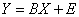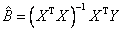Home News About Me Ph. D. Thesis Abstract Table of Contents 1. Introduction 2. Theory  Fundamentals of the Multivariate Data Analysis 2.1. Overview of the Multivariate Quantitative Data Analysis 2.2. Experimental Design 2.3. Data Preprocessing 2.4. Data Splitting and Validation 2.5. Calibration of Linear Relationships 2.5.1. PLS 2.6. Calibration of Nonlinear Relationships 2.7. Neural Networks  Universal Calibration Tools 2.8. Too Much Information Deteriorates Calibration 2.9. Measures of Error and Validation 3. Theory  Quantification of the Refrigerants R22 and R134a: Part I 4. Experiments, Setups and Data Sets 5. Results  Kinetic Measurements 6. Results  Multivariate Calibrations 7. Results  Genetic Algorithm Framework 8. Results  Growing Neural Network Framework 9. Results  All Data Sets 10. Results  Various Aspects of the Frameworks and Measurements 11. Summary and Outlook 12. References 13. Acknowledgements Publications Research Tutorials Downloads and Links Contact Search Site Map Print this Page## 2.5.   Calibration of Linear Relationships

For multivariate calibration of the concentrations of one or more analytes (variables y) and the device responses (independent variables x) the simplest and the most common approach is the assumption of a linear relationship. A model for this linear relationship can be setup by a multiple linear regression (MLR), which is also known as inverse least-squares regression (ILS). This calibration can be seen as an extension of the univariate linear regression. For each response variable, a linear equation is formulated:(5)

with bij as regression coefficients and ej as residuals. Using matrix notation equation (5) can be expressed as:(6)

The regression coefficients can be estimated by(7)

For the inversion of the variance-covariance matrix at least as many samples as device responses have to be measured. This inversion of the variance-covariance matrix of the independent variables, which is needed not only by MLR but also by most linear regression techniques to identify the model parameters, causes several problems. If the variables show a collinear behavior or if the variables are highly correlated, the resulting variance-covariance matrix will be ill-conditioned leading to unreliable model parameters und thus producing unstable calibration models.

Several methods have been developed to overcome these problems like principal component regression (PCR), partial least squares regression (PLS or PLSR), ridge regression (RR) and many more. Among these methods, "PLSR is the de facto standard for constructing a multivariate model" .

 Page 40 © Frank Dieterle, 03.03.2019